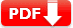# Lines (Geometry) Practice Test Question Answers

Two angles are called adjacent angles if they have a common vertex and a common arm but no common interior points. A linear pair is a pair of adjacent angles whose non-common sides are opposite rays.  When two lines intersect, the vertically opposite angles so formed are equal.

If two parallel lines are intersected by a transversal, (i) each pair of corresponding angles is equal. (ii) each pair of alternate interior angles is equal. (iii) each pair of interior angles on the same side of the transversal is
supplementary..

## Lines (Geometry) Practice Test Question Answers

 Test Name Lines Test Prep Type of Question Multiple Choice Question Answers Subject Math Total Question 10 Test Type Sample / Mock Test Editable & Printable PDF / Doc YES (Download link is given below) Available of Answers YES Difficulty Level Elementary Geometry

### Lines (Geometry) Practice Test Question Answers

 1. If two lines are intersected by a transversal, then the number of pairs of interior angles on the same side of the transversal is 🔘 A. 0 🔘 B. 1 🔘 C. 2 🔘 D. 3 🔘 E. 4
 2.  In the given figure, find out which pair of lines are parallel.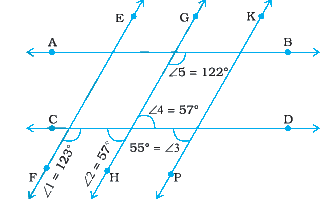🔘 A. GH || KP 🔘 B. EF || KP 🔘 C. AB || CD 🔘 D. EF || GH. 🔘 E. NOTA
Answer:D. EF || GH. [Solution: For lines EF and GH, taking CD as transversal, ∠1 and ∠2
are interior angles on the same side of the transversal. Therefore, we check whether the sum of ∠1 and ∠2 is 180° or
not. ∠1 = 123°, ∠2 = 57°, ∠1 + ∠2 = 123° + 57° = 180° Since the sum of the interior ∠’s on the same side of the
transversal is 180°, therefore EF || GH.
 3. In the following lines, l and m intersect each other at a point. Which of the following is false?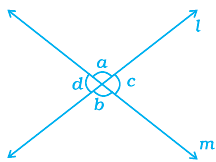🔘 A. ∠a = ∠b 🔘 B. ∠d = ∠c 🔘 C. ∠a + ∠d = 180° 🔘 D. ∠a = ∠d 🔘 E. NOTA
Answer:D ∠a = ∠d (Sol ∠a + ∠d = 180°)
 4.  In the following figure POR is a line. The value of a is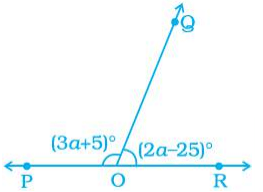🔘 A. 20° 🔘 B. 40° 🔘 C. 60° 🔘 D. 80° 🔘 E. 120°
Answer:B. 40° (Hints: (3a + 5)° + (2a – 25)° = 180°)
 5. In the following figure POQ is a line. If x = 30°, then ∠QOR is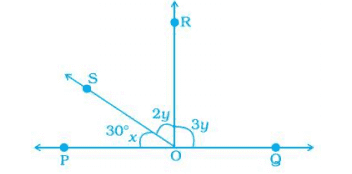🔘 A. 30° 🔘 B. 60° 🔘 C. 80° 🔘 D. 90° 🔘 E. 110°
 6. In the following figure, PA || BC || DT and AB || DC. Then, the values of a and b are respectively.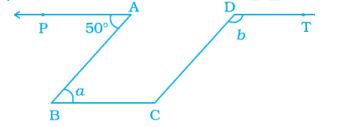🔘 A. 50°, 130° 🔘 B. 30°, 120° 🔘 C. 40°, 90° 🔘 D. 10°, 110° 🔘 E. 30°, 90°
Answer:A  50°, 130° (hints ∠ABC + ∠BCD = 180°)
 7.  In the following figure,  PQ || SR and SP || RQ. Then, angles a and b are respectively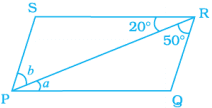🔘 A. 🔘 B. 🔘 C. 🔘 D. 🔘 E.
 8. In the following figure, lines AB and CD intersect at O. If ∠ AOC + ∠ BOE = 70° and ∠ BOD = 40°, find ∠ BOE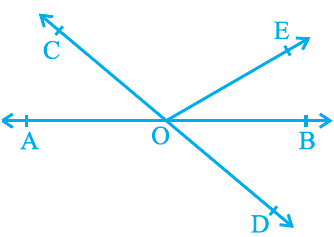🔘 A. 30° 🔘 B. 40° 🔘 C. 50° 🔘 D. 60° 🔘 E. 70°
 9. In the following figure, lines AB and CD intersect at O. If ∠ AOC + ∠ BOE = 70° and ∠ BOD = 40°, find ∠ COE🔘 A. 60° 🔘 B. 90° 🔘 C. 150° 🔘 D. 160° 🔘 E. 250°
 10. In the following figure, lines XY and MN intersect at O. If ∠ POY = 90° and a : b = 2 : 3, find c.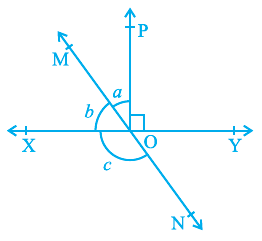🔘 A. 62° 🔘 B. 96° 🔘 C. 126° 🔘 D. 176° 🔘 E. 216°
 Document Type Download Link Free Editable Doc File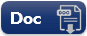Free Printable PDF File